# MATH 251: Calculus 3, SET8

## 16: Vector Calculus

### 16.3: The Fundamental Theorem for Line Integrals

These problems are done with the CAS. See Hand Solutions for details.

#### 1. [1094/3]

The vector field F is not conservative since it does not possess a potential function f; i.e., a function such that F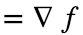, the gradient of f. In the code below, we use MATLAB's potential function to show this.
By hand, note that with F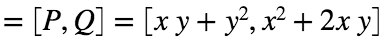we do not havefor these partial derivatives, which are continuous on the xy-plane. Thus by Theorem 5 on page 1090 of the textbook, F is not conservative.
syms x y real
P = x*y + y^2, Q = x^2 + 2*x*y
P =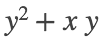Q =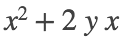F = [P Q]
F =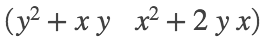f = potential(F, [x y]) % NaN or Not-a-Number means DNE (does not exist) in this context.
f = NaN
dP_dy = diff(P,y), dQ_dx = diff(Q,x)
dP_dy =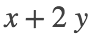dQ_dx =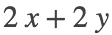#### 2. [1094/6]

The vector field F is conservative since it has a potential function f; i.e., a function such that F, the gradient of f.
(Again, see Hand Solutions for a check that this is true and how to actually construct the potential function by hand.)
syms x y
F = [y*exp(x) exp(x)+exp(y)]
F =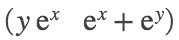f = expand( potential(F, [x y]) )
f =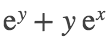#### 3. [1094/8]

syms x y
F = [2*x*y + y^(-2) x^2 - 2*x*y^(-3)]
F =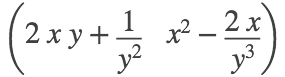f = expand( potential(F, [x y]) ) % potential function f
f =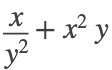#### 4. [1094/10]

syms x y
F = [log(y) + y/x log(x) + x/y]
F =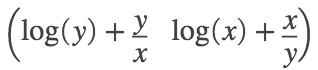f = expand( potential(F, [x y]) ) % potential function f
f =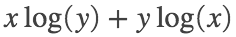#### 5. [1094/12]

The path C is the arc of the hyperbolafrom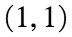to. The line integral of F along this path may be computed by the Fundamental Theorem for Line Integrals (FTLI) once we find a potential function f.
syms x y
F = [3 + 2*x*y^2 2*x^2*y]
F =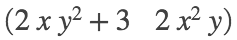% (a)
f(x,y) = expand( potential(F, [x y]) ) % potential function f
f(x, y) =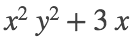% (b)
I = f(4,1/4) - f(1,1)
I = 9

#### 6. [1094/14]

The path C is the arc of the ellipse r, from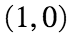to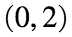. The line integral of F along this path may be computed by the Fundamental Theorem for Line Integrals (FTLI) once we find a potential function f.
syms x y
F = exp(x*y) * [1+x*y x^2]
F =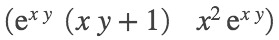% (a)
f(x,y) = expand( potential(F, [x y]) ) % potential function f
f(x, y) =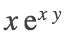% (b)
I = f(0,2) - f(1,0)
I =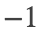#### 7. [1095/16]

The path C is the space curve r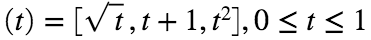, from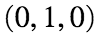to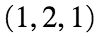. The line integral of F along this path may be computed by the Fundamental Theorem for Line Integrals (FTLI) once we find a potential function f.
syms x y z
F = [y^2*z + 2*x*z^2 2*x*y*z x*y^2 + 2*x^2*z]
F =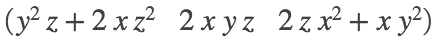% (a)
f(x,y,z) = expand( potential(F, [x y z]) ) % potential function f
f(x, y, z) =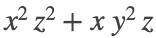% (b)
I = f(1,2,1) - f(0,1,0)
I = 5

#### 8. [1095/18]

The path C is the space curve r, from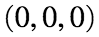to. The line integral of F along this path may be computed by the Fundamental Theorem for Line Integrals (FTLI) once we find a potential function f.
syms x y z
F = [sin(y) x*cos(y) + cos(z) -y*sin(z)]
F =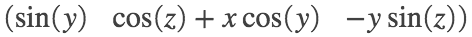% (a)
f(x,y,z) = expand( potential(F, [x y z]) ) % potential function f
f(x, y, z) =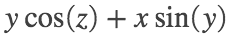% (b)
I = f(1,pi/2,pi) - f(0,0,0)
I =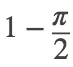I_appx = double(I)
I_appx = -0.5708

#### 9. [1095/20]

Here C is any path in the plane from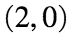to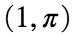. The line integral of F along this path may be computed by the Fundamental Theorem for Line Integrals (FTLI) once we find a potential function f.
syms x y
F = [sin(y) x*cos(y) - sin(y)]
F =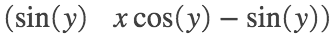% (a)
f(x,y) = expand( potential(F, [x y]) ) % potential function f
f(x, y) =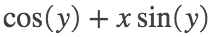% (b)
I = f(1,pi) - f(2,0)
I =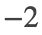#### 10. [1095/24]

Work is computed via the FTLI, analogous to #9.
syms x y
F = [2*x+y x]
F =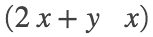% (a)
f(x,y) = expand( potential(F, [x y]) ) % potential function f
f(x, y) =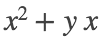% (b)
work = f(4,3) - f(1,1)
work = 26